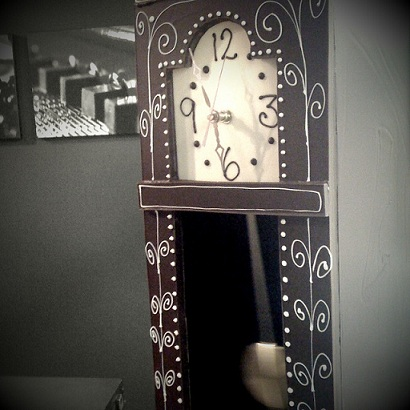# Difference Between Simple Harmonic Motion and Periodic Motion

## Main Difference – Simple Harmonic Motion vs. Periodic Motion

Simple harmonic motion is an important topic in the study of mechanics. The main difference between simple harmonic motion and periodic motion is that periodic motion refers to any type of repeated motion whereas simple harmonic motion (SHM) refers to a specific type of periodic motion where the restoring force is proportional to the displacement.

## What is Simple Harmonic Motion

Any type of repeated motion requires a force. This is because any type of repeated motion involves a change in direction. To change the direction of an object, a force is required. The simple harmonic motion refers to types of repeated motion where the restoring force that keeps objects moving repetitively is proportional to the displacement of the object.

If a mass is hung on a spring and pulled down slightly, the mass would start moving up and down periodically. In this case, the motion is a type of simple harmonic motion. This means that during the motion, the displacement of the mass ($x$) is proportional to the restoring force$F$:$F\propto -x$

The negative sign here indicates that the restoring force is always in a direction opposite to the direction of displacement. In other words, the restoring force is always trying to pull the mass towards the equilibrium position. By introducing a constant$k$, write down the above relationship as an equation:$F=-kx$

The period of simple harmonic motion (how long it takes for the mass to complete one full cycle of motion, given by$T$) can be shown to be:$T=2\pi \sqrt{\frac{m}{k}}$

where$m$ refers to the mass of the object.

A simple pendulum oscillating through a small angle is also in simple harmonic motion. If the pendulum has a length$l$, the period of its simple harmonic motion is given by:$T=2\pi \sqrt{\frac{l}{g}}$

where$g$ is the acceleration due to gravity.A pendulum in a grandfather clock undergoes simple harmonic motion.

## What is Periodic Motion

Periodic motion refers to any type of repeated motion. In this sense, simple harmonic motion is also a special case of periodic motion. That is, any simple harmonic motion (SHM) is periodic. Because if there is no force acting against the SHM, it will not subject to a damping (damping motion is not SHM). So, it is a repeating or periodic motion. But the inverse of this is not always true. So, there can be periodic motions that are not simple harmonic (circular motion is periodic but not simple harmonic). For instance, the motion of an object in a circle at constant speed is a periodic motion but not a simple harmonic one. However, if you look at the projection of a particle in uniform circular motion onto the diameter of the circular path, this projection’s motion can be described as simple harmonic motion.

## Difference Between Simple Harmonic Motion and Periodic Motion

Periodic motion refers to any type of repeated motion.

Simple harmonic motion refers to types of periodic motion where there is a restoring force which is proportional to the displacement.

Image Courtesy:

“Working Grandfather Clock” by Emily McCracken (Own work) [CC BY-SA 2.0], via flickr